##### Geometry Workbook For Dummies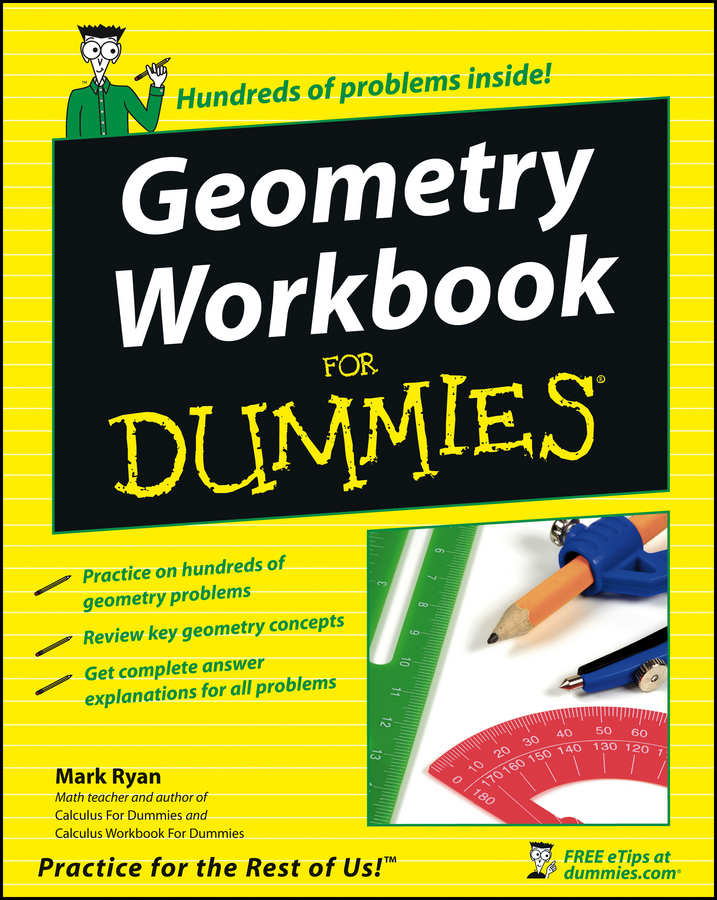Definitions, theorems, and postulates are the building blocks of geometry proofs. With very few exceptions, every justification in the reason column is one of these three things. The below figure shows an example of a proof.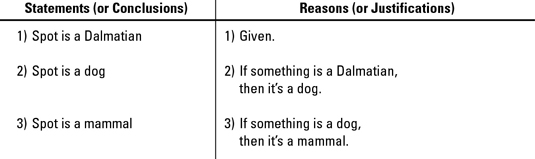If this had been a geometry proof instead of a dog proof, the reason column would contain if-then definitions, theorems, and postulates about geometry instead of if-then ideas about dogs. Here’s the lowdown on definitions, theorems, and postulates.

## Using definitions in the reason column

Definition: A definition defines or explains what a term means. Here’s an example: “A midpoint divides a segment into two congruent parts.”

You can write all definitions in if-then form in either direction: “If a point is a midpoint of a segment, then it divides that segment into two congruent parts” or “If a point divides a segment into two congruent parts, then it’s the midpoint of that segment.”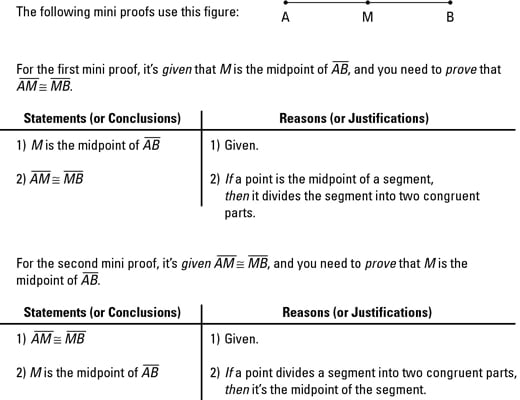The above figure shows you how to use both versions of the midpoint definition in a two-column proof.

When you have to choose between these two versions of the midpoint definition, remember that you can think of the word if as meaning because I already know and the word then as meaning I can now deduce. For example, for reason 2 in the first proof in the figure, you choose the version that goes, “If a point is the midpoint of a segment, then it divides the segment into two congruent parts,” because you already know that M is the midpoint of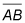(because it’s given) and from that given fact you can deduce that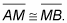## Using theorems and postulates in the reason column

Theorem and postulate: Both theorems and postulates are statements of geometrical truth, such as All right angles are congruent or All radii of a circle are congruent. The difference between postulates and theorems is that postulates are assumed to be true, but theorems must be proven to be true based on postulates and/or already-proven theorems. This distinction isn’t something you have to care a great deal about unless you happen to be writing your Ph.D. dissertation on the deductive structure of geometry. However, because you’re probably not currently working on your Ph.D. in geometry, you shouldn’t sweat this fine point.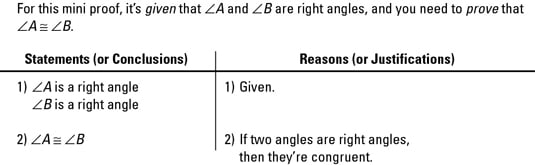Written in if-then form, the theorem All right angles are congruent would read, “If two angles are right angles, then they’re congruent.” Unlike definitions, theorems are generally not reversible. For example, if you reverse this right-angle theorem, you get a false statement: “If two angles are congruent, then they’re right angles.” (If a theorem works in both directions, you’ll get a separate theorem for each version. The two isosceles-triangle theorems — If sides, then angles and If angles, then sides — are an example.) The above figure shows you the right-angle theorem in a proof.

When you’re doing your first proofs, or later if you’re struggling with a difficult one, it’s very helpful to write your reasons (definitions, theorems, and postulates) in if-then form. When you use if-then form, the logical structure of the proof is easier to follow. After you become a proof expert, you can abbreviate your reasons in non-if-then form or simply list the name of the definition, theorem, or postulate.